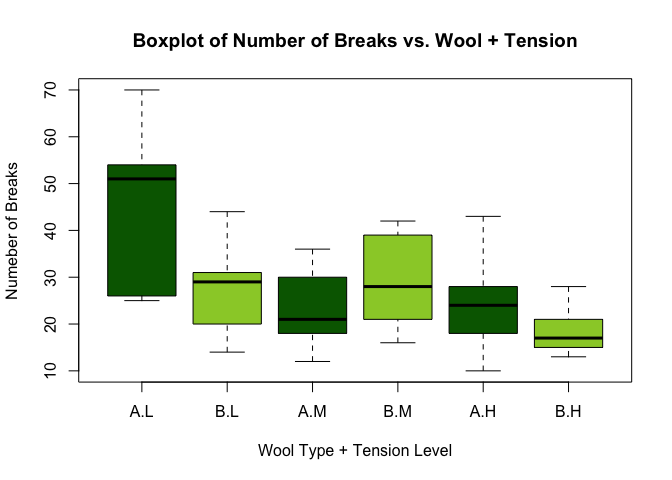# Exercise 1: Graphs

For this exercise, we will use practice plotting different types of graphs on multiple built-in datasets in R.

## PlantGrowth

• Take a look at the PlantGrowth dataset by typing it in the console.
• Use the table() function to get counts on the number of plants in each treatment groups.
• Your output should be as followed
table(PlantGrowth$group) ## ## ctrl trt1 trt2 ## 10 10 10 ### Histogram • Plot one histogram of dried weigth (weight) of plants from all 3 treatment groups. • Your histogram should look similar (might not be exactly the same) to the plot below. hist(x = PlantGrowth$weight, probability = TRUE, border = "dodgerblue",
main = "Histogram of the Plants' Dried Weight",
xlab = "Dried Weight (lbs.)", breaks = 10)
grid()
box()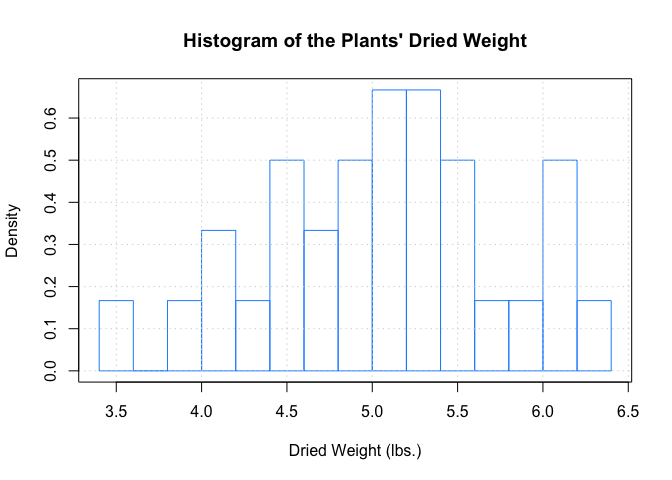### Boxplot

• To compare the treatments, we want to have a plot that can compare the dried weight of plants from each treatment group.
• Plot a boxplot of Weight vs. Group.
• Your plot should look like this:
boxplot(formula = weight ~ group, data = PlantGrowth,
main = "Boxplot of Plants' Dried Weights vs. Treatments",
ylab = "Dried Weight (lbs.)", xlab = "Treatment Group",
col = c("darkorange", "dodgerblue", "lightgrey"))
grid()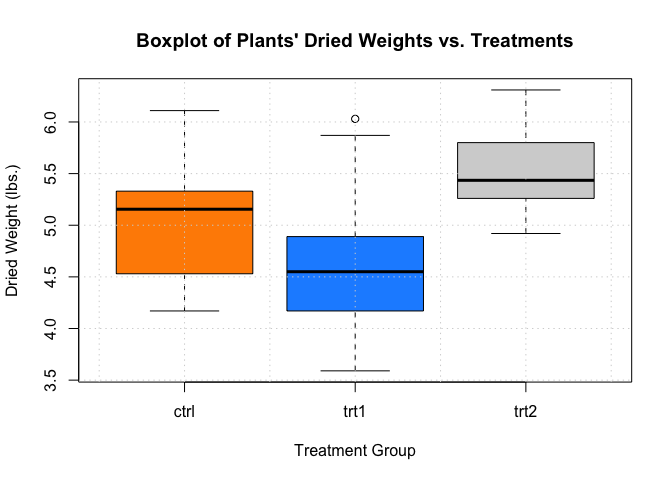## trees

• Next, we’re taking a look at the trees dataset.

### Scatterplot

• Plot a scatterplot of Height vs. Girth (tree diameter).
• An example plot is shown below.
plot(formula = Height ~ Girth, data = trees,
main = "Black Cherry Trees Girth vs Height",
xlab = "Girth (in)", ylab = "Height (ft)",
col = "dodgerblue", pch = 19)
grid()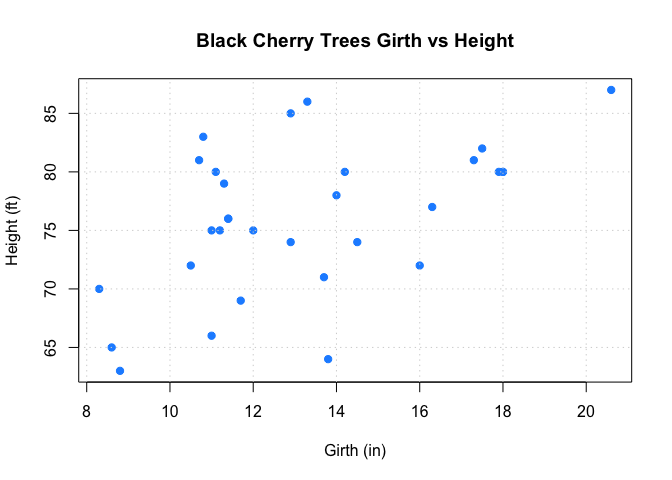### Boxplot

• To get summary statistics from a vector (or a column of a data frame, which is a vector), we can use fivenum().
• For example, the five main summary statistics of Black Cherry Trees’ Girth are
fivenum(trees$Girth) ##  8.30 11.05 12.90 15.25 20.60 • That means: min = 8.3, q1 = 11.05, median = 12.9, q3 = 15.25, max = 20.6. • We will use these numbers to divide the dataset into 5 groups based on their Girth measurement. Create a new column Group in trees that contain the following value: • group1: if the girth is in [8.3, 11.05) • group2: [11.05, 12.90) • group3: [12.90, 15.25) • group4: [15.25, 20.60] • So how do we do that in R? • We can use a function called cut(). Google this function or look at the documentation by typing ?cut in the RStudio console. • The data frame trees should look like this after you add the Group column. trees$Group <- cut(trees$Girth, breaks = fivenum(trees$Girth),
labels = c("group1", "group2", "group3", "group4"),
right = FALSE, include.lowest = TRUE)
trees
##    Girth Height Volume  Group
## 1    8.3     70   10.3 group1
## 2    8.6     65   10.3 group1
## 3    8.8     63   10.2 group1
## 4   10.5     72   16.4 group1
## 5   10.7     81   18.8 group1
## 6   10.8     83   19.7 group1
## 7   11.0     66   15.6 group1
## 8   11.0     75   18.2 group1
## 9   11.1     80   22.6 group2
## 10  11.2     75   19.9 group2
## 11  11.3     79   24.2 group2
## 12  11.4     76   21.0 group2
## 13  11.4     76   21.4 group2
## 14  11.7     69   21.3 group2
## 15  12.0     75   19.1 group2
## 16  12.9     74   22.2 group3
## 17  12.9     85   33.8 group3
## 18  13.3     86   27.4 group3
## 19  13.7     71   25.7 group3
## 20  13.8     64   24.9 group3
## 21  14.0     78   34.5 group3
## 22  14.2     80   31.7 group3
## 23  14.5     74   36.3 group3
## 24  16.0     72   38.3 group4
## 25  16.3     77   42.6 group4
## 26  17.3     81   55.4 group4
## 27  17.5     82   55.7 group4
## 28  17.9     80   58.3 group4
## 29  18.0     80   51.5 group4
## 30  18.0     80   51.0 group4
## 31  20.6     87   77.0 group4
• Now, plot a boxplot of Height vs. Group.
• Your plot should look similar to this.
boxplot(formula = Height ~ Group, data = trees,
main = "Boxplot of Girth Group vs. Height",
xlab = "Girth Group", ylab = "Height (ft)",
col = c("purple", "pink", "violet", "lightgrey"))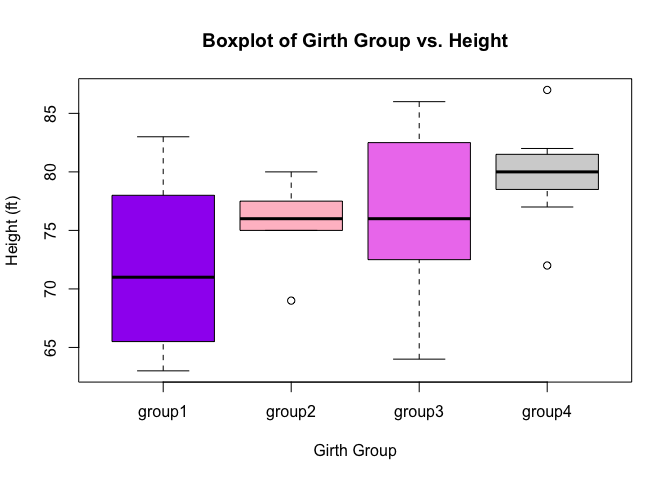## warpbreaks

• Use str() to take a quick look at the warpbreaks dataset.
• Use table() to count how many experiments were done for each combination of wool and tension.
• Your output should be as followed.
##     tension
## wool L M H
##    A 9 9 9
##    B 9 9 9

### Histogram

• Plot a histogram of the number of breaks breaks for all combination of wool and tension.
• Your graph should look similar to the plot shown below.
hist(x = warpbreaks\$breaks, main = "Histogram of Number of Breaks",
probability = TRUE, breaks = 10,
xlab = "Number of Breaks", border = "darkorange")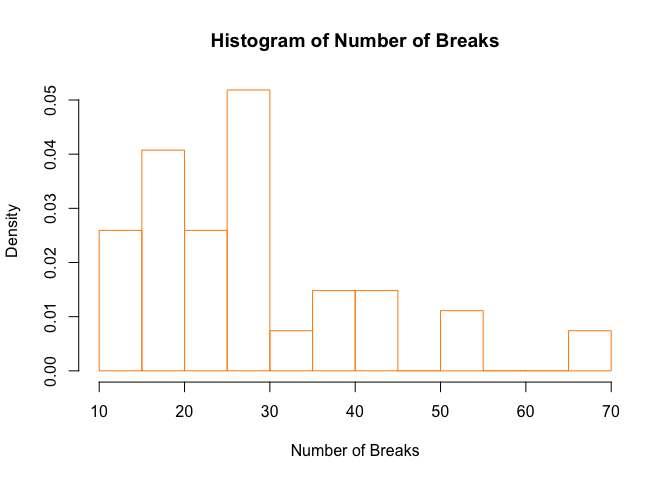### Boxplot

• Plot a boxplot of breaks vs. tension.
• Your plot might look like this.
boxplot(formula = breaks ~ tension, data = warpbreaks,
main = "Boxplot of Number of Breaks vs. Tension Level",
xlab = "Tension Level", ylab = "Numeber of Breaks",
col = c("darkred", "red", "darkorange"))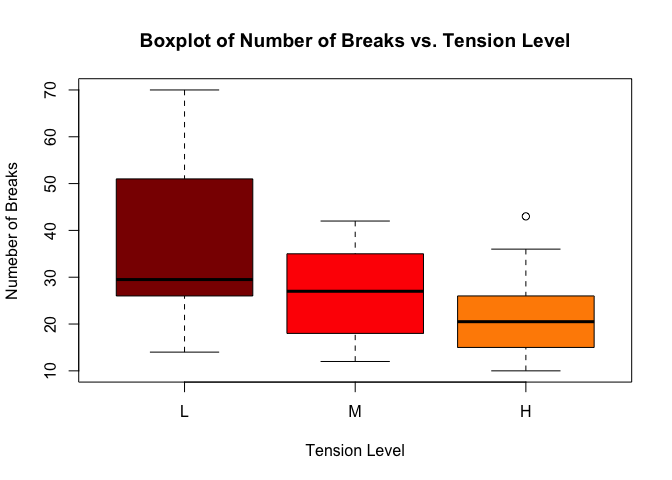### Another Boxplot

• Plot a boxplot of breaks vs. wool.
• Your plot might look like this.
boxplot(formula = breaks ~ wool, data = warpbreaks,
main = "Boxplot of Number of Breaks vs. Wool Type",
xlab = "Wool Type", ylab = "Numeber of Breaks",
col = c("darkgreen", "yellowgreen"))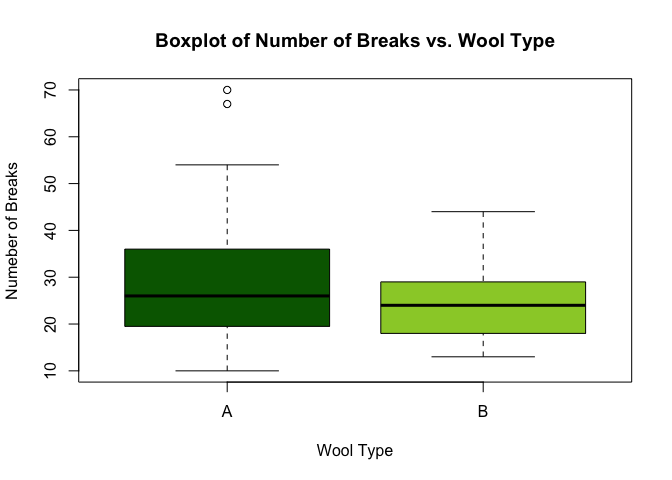### Another Boxplot (Again!)

• Now, plot a boxplot of breaks vs. combination of wool and tension (hint: wool*tension).
boxplot(formula = breaks ~ wool*tension, data = warpbreaks,
main = "Boxplot of Number of Breaks vs. Wool + Tension",
xlab = "Wool Type + Tension Level", ylab = "Numeber of Breaks",
col = c("darkgreen", "yellowgreen"))